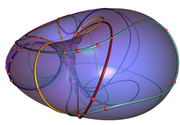max planck institut
informatik# Numerical Algebraic Computation – Winter Term 2013/2014

## News:

• Exercises take place at Tuesday 2-4pm, in Room 23, Campus E1.4 (MPI for Computer Science)

## Lectures

Date Topic Reference Homework
Oct 16 Overview&Motivation ReliableComputing.pdf (Rump) no exercise
Oct 23 Floating Point Arithmetic and Error Analysis numbers.pdf (Mehlhorn), cp.pdf (Mehlhorn, Sagraloff) Ex01.pdf
Oct 30 Box Functions, Interval Arithmetic and a First Application SoftPredicate.pdf (Yap et al.) Ex02.pdf

## Overview:

We will give an introduction in the area of numerical algebraic computation, where the focus is on reliable methods which use approximate but certified arithmetic. For some methods, we will also study their worst case complexity and show that the use of approximate arithmetic instead of exact arithmetic yields better asymptotic bounds. Possible topics are:

• basic integer and polynomial arithmetic: representation, school method vs. faster methods for multiplication, floating point arithmetic, interval arithmetic, division with remainder, (multipoint-) evaluation.
• (polynomial) root finding: numerical complex solvers (e.g.~Durand Kerner method, Laguerre's method), real root solvers (Newton's method, Descartes method), analytic functions.
• Matrix computations: singularity of a matrix, determinant computation, linear system solving.
• polynomial system solving: hybrid methods based on elimination techniques, homotopy methods.

## Basics and Dates:

• Time: Wednesday, 2 – 4 c.t.
• Room: Campus E1.4, seminar room 024
• Lecturers and office hours: Michael Sagraloff (Thursday 10 – 11)
• First lecture: Wednesday, October 16 (Campus E1 4, 024)
• Exercises(s): Time will be fixed in the first lecture.

## Prerequisites:

• Analysis I-II and Linear Algebra I-II or Mathematics for Computer Scientists I and II
• Basic knowledge in Number Theory, Algebra, and Complex Analysis is useful, but not required.
• Programming experience in Maple (or another computer algebra system) is useful, but not required.

## Information and Rules:

This is an advanced course for students in computer science and mathematics.
Successful completion (that is, solving the expected 50 % of the weekly exercises and passing the exam) is worth 6 ECTS credit points. Expect to be imposed a total workload of about 140 hours, distributed to 45 hours of attended lectures and 95 hours of private study.

This course is intended for graduate students and/or senior undergraduate students. It consists of one two-hour lecture and a two-hour tutorial session per week.

The grade is determined by the final exam (oral or written).

## Exercises:

• There will be theoretical and practical (i.e., programming) exercises, to be handed in before the lecture in the lecture hall.
• You need to attend at least 75% of all lectures and tutorials. This is a relaxed but strict rule and no negotiation is possible.
• You need to obtain at least 50% of the total exercise scores.
• Every student has to present the solution to two assignments (typically about 3 per sheet) in the tutorial sessions.
• Every student has to prepare "LaTeX'ed" (sources and pdf) sample solutions to one assignment sheet. Sample solutions are due one week after discussing them in the tutorial. Submitted sample solutions will be checked, and we may ask for improvements.